# MCAT Physical : Photons and Photon Energy

## Example Questions

### Example Question #1 : Photons And Photon Energy

The electron shell energies of a particular atom are given below: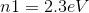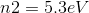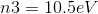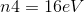Which of the following colors can this atom emit when an electron falls from a higher to lower energy shell?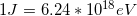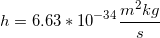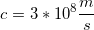Green

Red

Orange

Yellow

Violet

Violet

Explanation:

We can see that eV differences between the shells for this atom range anywhere from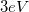(between n2 and n1) to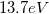(between n4 and n1). We can use these two values to find the range of wavelengths that can be produced by photons from this atom. To find the wavelength, we will use the formula:In this equation,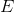is the energy in Joules,is Planck's constant,is the speed of light, and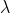is the wavelength.

First, convert the energy changes to Joules using the given conversion factor.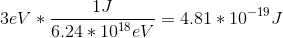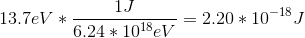Use these energies in the first equation to solve for the range of possible wavelengths.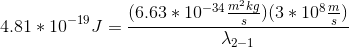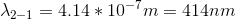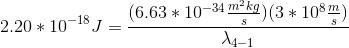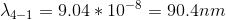The visible spectrum spans from approximately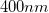to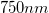, with smaller wavelengths corresponding to violet and blue and larger wavelengths corresponding to red. Since the range of possible wavelengths only overlaps with a small portion of the visible spectrum, we can see that the only color that can be generated when an electron falls from a higher level to a lower level is violet. The other transitions will generate photons in the ultraviolet range.

### Example Question #1 : Photons And Photon Energy

Light is passed through a prism to separate the wavelengths, and red, green and blue portions of the spectrum are sequentially projected onto a photo-electric surface connected to an electrical circuit. Which of these observations is correct?

1. Red light, but not green or blue light, will generate an electric current.
2. Red light and blue light, but not green light, will generate an electric current.
3. Green light, but not red or blue light, will generate an electric current.
4. Blue light, but not red or green light, will generate an electric current.
5. All the frequencies will generate an electric current if the intensity is adequate.

5

3

2

4

1

4

Explanation:

Choice 4 is correct because blue light is much more energetic than red light. The most intense low-frequency light will not generate any current at all, because the wavelength is not sufficiently energetic to displace electrons from the photo-electric surface; however, once it is established that a certain frequency is capable of generating current, then the amount of current is dependent upon intensity.

Mnemonic:  “Blue is better.”

### Example Question #31 : Waves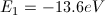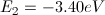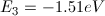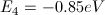The first four energy levels of a hydrogen atom have the energies given above. If a hydrogen atom is initially in the n = 2 state, photons of which of the following energies could be absorbed?

1.89, 2.55, or 10.2eV

1.89eV or 2.55eV

10.2eV only

1.89eV or 10.2eV

1.89eV only

1.89eV or 2.55eV

Explanation:

Absorbing a photon would have the effect of pushing the atom into a higher energy state, in this case n = 3 or n = 4. Photons with an energy equal to the difference betweeen E2 and E3 or between E2 and E4, could be absorbed.

E3 – E2 = –1.51 – (–3.40) = 1.89eV

E4 – E2 = –0.85 – (–3.40) = 2.55eV

### Example Question #4 : Photons And Photon Energy

A student wishes to run an experiment that requires photons with energy between 12.5eV and 13.0eV per photon. The light source is a hydrogen lamp. Which hydrogen electron transition would produce an appropriate photon?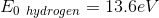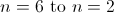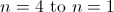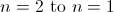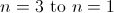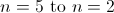Explanation:

To calculate photon energy from an electron transition, we use the following equation.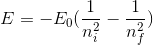In the formula,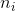is the initial energy level and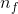is the final energy level.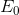is a constant for the given compound. Our first step will to find the difference described in the formula using the constant given for hydrogen and an estimate for the energy produced.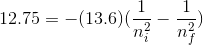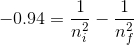We can use guess and check to estimate the discrete values that can be used for the electron energy levels.

We find that if the initial energy level is 4 and the final energy level is 1, the value of the difference is approximately -0.94.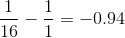An electron transition from energy level 4 to energy level 1 would produce a photon in the appropriate range.

### Example Question #5 : Photons And Photon Energy

In the photoelectric effect experiment, incoming photons with enough energy cause the ejection of an electron from a metal plate, proving that light carries energy. Which color of visible light would you expect to produce the fastest ejected electrons?

Yellow

Green

Red

Orange

Blue

Blue

Explanation:

This question can be approached in two ways. The first way is to have a general understanding of the photoelectric effect, and its equation: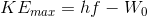, where the kinetic energy of an ejected electron is equal to difference between the energy of the incoming photon, and the work function of the metal plate. If the energy of the photon is greater than that of the work function, an electron will be emitted with a speed that is proportional that difference, thus the greater the energy of the photon, the greater the total kinetic energy, and the faster the speed of the outgoing electron.

Since wavelength is inversely proportional to energy, and because we know that blue light has a wavelength around 400nm, and red light approximately 700nm, we would expect blue light to carry the most energy and thus result in the fastest ejected electron.

A second approach to this question is to use critical reasoning. Using the concept of energy conservation, we can predict the energy of the incoming photon will be transferred to the outgoing electron. Because we know that energy is inverse to wavelength, the lowest wavelength photon will have the most energy. Applying conservation of energy principles and the fact that energy is directly proportional to velocity, it is a good assumption to reason that the lowest wavelength photon will create the highest velocity electron. This leads to the answer of blue light.

### Example Question #1 : Photons And Photon Energy

Which of the following electron transitions in a hydrogen atom would emit a photon of the lowest frequency?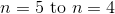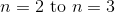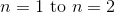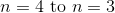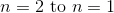Explanation:

A photon is emitted if the electron goes from a higher to lower energy level, so we need a choice where the energy level, n, decreases. Also, we need to look for the transition that has the smallest energy difference, since frequency is proportional to energy (f  = E/h, where h is Planck's constant). Higher energy levels are closer together, so the highest pair of levels has the smallest difference in energy and the lowest frequency of emitted photons.

### Example Question #7 : Photons And Photon Energy

A sodium lamp emits a yellow light with a frequency of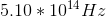. How much energy is contained in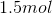of photons?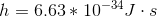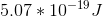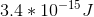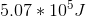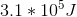Explanation:

The energy of a single photon is given by the equation: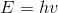We are given the frequency and the value of the constant, allowing us to solve.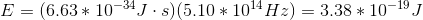The above gives the energy contained in one photon. Next, solve for the energy contained inusing Avogadro's number: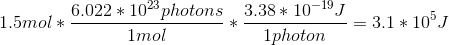### Example Question #8 : Photons And Photon Energy

An incandescent light bulb is shown through a glass prism. The certain wavlength of the light is then directed into a glass cuvette containing an unknown concentration of protein. Commonly, this process is called spectroscopy and is used to determine the concentrations of DNA, RNA, and proteins in solutions. The indices of reflection of air, glass, and the solution are 1, 1.5, and 1.3, respectively.

How much energy does a photon of red light with a wavelength of 690nm traveling through the solution contain?

2.19 * 10-19J

7.43 * 10-19J

5.25 * 10-19J

3.30 * 10-19J

2.19 * 10-19J

Explanation:

This question asks us about the particle nature of light and how much energy a photon would contain. From our light equations, we know that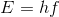, where E is the energy of a single photon, h is Plank’s constant, and f is the frequency of the photon.

From the information in the problem, we need to determine the frequency.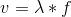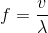We need to determine the velocity of the light in the solution. We can use the definition of index of refraction to determine this value, along with the speed of light and the index of refraction of the solution.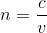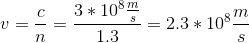Now we can compute the frequency.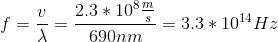Substituting the frequency we found, along with Plank’s constant, we can find the energy.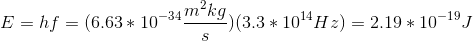### Example Question #9 : Photons And Photon Energy

What color of visible light contains enough energy to eject an electron off of a metal with a work function of?Red

Yellow

Violet

Orange

Green

Violet

Explanation:

Work function is the minimum amount of energy, in electron-volts, required to remove an electron from a metal surface. To calculate the energy of a single photon of light, one must use the equation:In this formula,is energy in Joules,is Planck's Constant, andis the speed of light.

To convert from Joules to eV, one must use the given conversion factor.Use this value in the energy formula to find the wavelength of the light.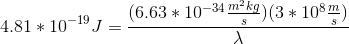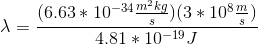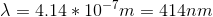The visible spectrum spans from approximatelyto, with smaller wavelengths corresponding to violet and blue and larger wavelengths corresponding to red. Even without knowing the exact wavelength correlations in the spectrum, we know that our wavelength is very small and will be found in the violet end of the spectrum.